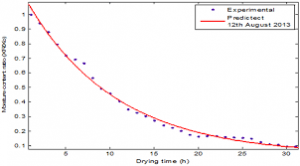## How to Calculate and Solve for Dryer Capacity | Polymer & TextileThe image above represents dryer capacity.

To compute for dryer capacity, two essential parameters are needed and these parameters are material consumption (Mcand drying time (TD).

The formula for calculating dryer capacity:

Dc = Mc / TD

Where:

Dc = Dryer Capacity
Mc = Material Consumption
TD = Drying Time

Let’s solve an example;
Given that the material consumption is 28 and the drying time is 34. Find the dryer capacity?

This implies that;

Mc = Material Consumption = 28
TD = Drying Time = 34

Dc = Mc / TD
Dc = 28 / 34
Dc = 0.82

Therefore, the dryer capacity is 0.82.

Calculating for Material Consumption when the Dryer Capacity and the Drying time is Given.

Mc = Dc x TD

Where:

Mc = Material Consumption
Dc = Dryer Capacity
TD = Drying Time

Let’s solve an example;
Find the material consumption when the dryer capacity is 40 and the drying time is 2.

This implies that;

Dc = Dryer Capacity = 40
TD = Drying Time = 2

Mc = Dc x TD
Mc = 40 x 2
Mc = 80

Therefore, the material consumption is 80.
Continue reading How to Calculate and Solve for Dryer Capacity | Polymer & Textile